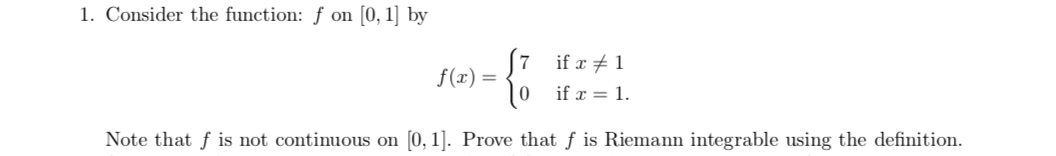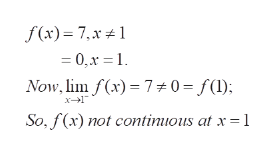1. Consider the function: f on [0, 1] by7 if r1f(x)|0 if 10, 1]. Prove that f is Riemann integrable using the definitionNote that f is not continuous on

Questionhelp_outlineImage Transcriptionclose1. Consider the function: f on [0, 1] by 7 if r1 f(x) |0 if 1 0, 1]. Prove that f is Riemann integrable using the definition Note that f is not continuous on fullscreen
Step 1

To prove that f is not continuous but Riemann integrable

Step 2

f(x) is not continuous at x=1 as the left hand side limit of f(x) as x approaches 1 is not equal to the value f(1).help_outlineImage Transcriptionclosef (x)= 7,x 1 = 0,x = 1 Now, lim f(x)70 = f() x1 So, f(x) not contimious at x 1 fullscreen
Step 3

The discontinuity can also be proved by observing the graph of t...

Want to see the full answer?

See Solution

Want to see this answer and more?

Our solutions are written by experts, many with advanced degrees, and available 24/7

See Solution
Tagged in

Math